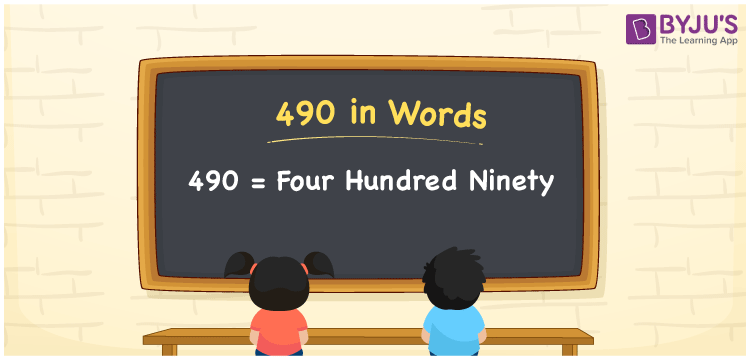# 490 in Words

490 in words is written as Four hundred ninety. In both the International System of Numerals and the Indian System of Numerals, 490 is written as Four hundred ninety. The number 490 is a Cardinal Number as it represents some quantity. For example, “Susan scored 490 in the exams”, 490 quantifies the total score.

 490 in Words Four hundred ninety Four hundred ninety in Number 490

## 490 in English Words

We write 490 in English Words using the letters of English alphabets. Therefore, we read 490 in English as “Four hundred ninety”.## How to Write 490 in Words?

To write 490 in words, we shall use the place value chart. In the place value chart, write 0 in the ones, 9 in the tens, and 4 in the hundreds. Now let us make a place value chart to write the number 490 in words.

 Hundreds Tens Ones 4 9 0

Thus, we can write the expanded form as

4 × Hundred + 9 × Ten + 0 × One

= 4 × 100 + 9 × 10 + 0 × 1

= 400 + 90 + 0

= 490

= Four hundred ninety.

490 is a natural number which is the successor of 489 and the predecessor of 491.

490 in words – Four hundred ninety

• Is 490 an odd number? – No
• Is 490 an even number? – Yes
• Is 490 a perfect square number? – No
• Is 490 a perfect cube number? – No
• Is 490 a prime number? – No
• Is 490 a composite number? – Yes

## Frequently Asked Questions on 490 in Words

Q1

### How to write 490 in words?

490 in words is written as Four hundred ninety.
Q2

### How to write 490 in words in the International and Indian System of Numerals?

In both the system of numerals, 490 is written as Four hundred ninety.
Q3

### How to write 490 in a place value chart?

In the place value chart, write 0 in the ones, 9 in the tens, and 4 in the hundreds.